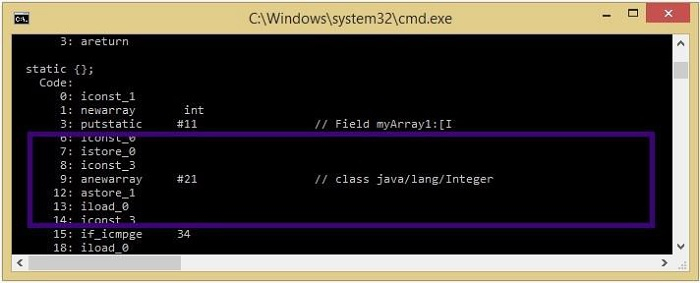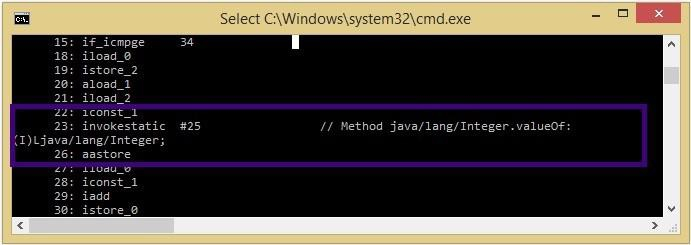# IntArray vs. Array in Kotlin

In this article, we will take an example to show the difference between IntArray and Array<Int>in Kotlin.

## IntArray in Kotlin

IntArray is a class in Kotlin representing the array of elements. Each instance of this class is represented as an integer array. To the constructor of this class you need to pass the number of elements you need in the array (size).

You can instantiate this class as shown below −

val myArray = IntArray(8)

By default, all the elements of the created array will be initialized to "0".

## Example

The following program creates an array of integers by instantiating the IntArray class −

fun main(args: Array<String>) {
// Creating an object of the IntArray class
val myArray = IntArray(3)

// Populating the array
myArray = 21;
myArray = 10;
myArray = 8;

// Converting the array to string
val res = myArray.joinToString()
println("Contents of the created array: "+res)
}

## Output

On executing the above program, it will generate the following output −

Contents of the created array: 21, 10, 8

## Array<Int> in Kotlin

Array<T> is a class in Kotlin which represents an array (that too, a native Java array). It is a generic class, i.e., you can specify the type (T) of the array to be created. Similar to the IntArray class, you need to pass the number of elements you need in the array (size). The statement Array<Int> in Kotlin creates an integer array. You can create an integer array using this class as shown below −

val myArray = Array(3) {0}

By default, all the elements of the created array will be initialized to "0".

## Example

The following program creates an array of integers by instantiating the Array class −

fun main(args: Array<String>) {
// Creating an object of the Array class
val myArray = Array(3) {0}

// Populating the array
myArray = 101;
myArray = 330;
myArray = 8;

// Converting the array to string
val res = myArray.joinToString()
println("Contents of the created array: "+res)
}

## Output

On executing the above program, it will generate the following output −

Contents of the created array: 101, 330, 8

## IntArray vs. Array<Int>

Internally, Array<Int> creates an array of type Integer. It is just like creating an instance of the Integer class in Java. Values created using this syntax will be boxed. Whereas, IntArray creates an array similar to a Java primitive array.

Consider the following example −

// Creating an integer array using the IntArray class
val myArray1 = IntArray(1)

// Creating an integer array using the Array class
val myArray2 = Array(3){1}

You can compile this line and verify the bytecode of this using the javap command as −

> kotlinc Test.kt
> javap -c -p Testkt

Once you do that for the first statement, you can observe that internally a simple array is created.Whereas for the second statement, you can observe that the array was translated to an array of java.lang.Integer (Integer[]).